TitleCollege Algebra
Answer/Discussion to Practice Problems
Tutorial 16: Formulas and Applications

WTAMU > Virtual Math Lab > College Algebra > Tutorial 16: Formulas and ApplicationsAnswer/Discussion to 1a In last night’s basketball game, Sally scored 9 less than twice what Lucy scored.  The sum of their scores is 27.  How many points did Sally and Lucy make individually?

 Step 1: Understand the problem.

 Make sure that you read the question carefully several times.  We are looking for two numbers, and since we can write the number of points Sally made in terms of the number of points Lucy scored we will let  x = the number of points Lucy scored   Sally scored 9 less than twice what Lucy scored: 2x - 9 = number of points Sally scored

 Step 2:  Devise a plan (translate).Step 3:  Carry out the plan (solve).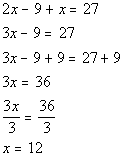*Combine like terms *Inv. of sub. 9 is add. 9 *Inv. of mult. by 3 is div. by 3

 If we add 12 and a 15 (a number 9 less than twice 12) we do get 27.     FINAL ANSWER: The number of points Lucy scored was 12. The number of points Sally scored was 15.Answer/Discussion to 1b The heights in inches of three basketball players are 3 consecutive integers.  If the sum of twice the 1st, 3 times the 2nd, and the 3rd is 437, what are the three heights.

 Step 1: Understand the problem.

 Make sure that you read the question carefully several times.  We are looking for 3 consecutive integers, we will let x = 1st consecutive integer x + 1 = 2nd consecutive integer x + 2  = 3rd  consecutive integer

 Step 2:  Devise a plan (translate).Step 3:  Carry out the plan (solve).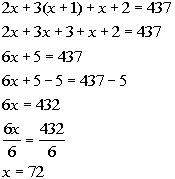*Remove ( ) by using dist. prop. *Combine like terms *Inv. of add. 5 is sub. 5   *Inv. of mult. by 6 is div. by 6

 If we take the sum of twice 72, three times 73, and 74, we do get 437     FINAL ANSWER: The heights of the three basketball players in inches are 72, 73, and 74.Answer/Discussion to 1c A rectangular floor has a perimeter of 54 feet.  If the length is 3 more than the width, what are the dimensions of the floor?

 Step 1: Understand the problem.

 Make sure that you read the question carefully several times.    We are looking for the length and width of the rectangle.  Since length can be written in terms of width, we will let w = width   length is 3 feet more than  the width: w + 3 = length

 Step 2:  Devise a plan (translate).Step 3:  Carry out the plan (solve).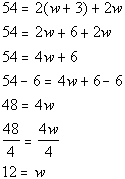*Remove ( ) by using dist. prop. *Combine like terms *Inv. of sub. 6 is add. 6 *Inv. of mult. by 4 is div. by 4

 If width is 12, then length, which is 3 feet more than the width, would have to be 15.  The perimeter of a rectangle with width of 12 feet and length of 15 feet does come out to be 54.   FINAL ANSWER: Width is 12 feet. Length is 15 feet.Answer/Discussion to 1d The original price of a CD player was marked down 15% and is now \$127.50, how much was the original price?

 Step 1: Understand the problem.

 Make sure that you read the question carefully several times.  We are looking for the price of the CD player before the markdown, we will let x = price of the CD player before the markdown.

 Step 2:  Devise a plan (translate).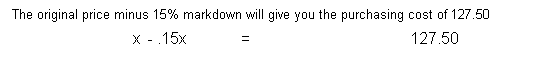Step 3:  Carry out the plan (solve).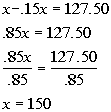*Combine like terms *Inv of mult. .85 is div. by .85

 If you markdown 15% from \$150 you would get \$127.50.     FINAL ANSWER: The original price is \$150.Answer/Discussion to 2afor T

 In this problem, we need to solve for T.  This means we need to get T on one side and EVERYTHING ELSE on the other side using inverse operations. Let’s solve this formula for T: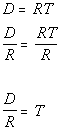*Inverse of mult. by R is div. by R       *Formula solved for TAnswer/Discussion to 2bfor m

 In this problem, we need to solve for m.  This means we need to get m on one side and EVERYTHING ELSE on the other side using inverse operations. Let’s solve this formula for m: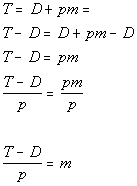*Inverse of add. by D is sub. by D *Inverse of mult. by p is div. by p     *Formula solved for m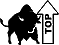WTAMU > Virtual Math Lab > College Algebra > Tutorial 16: Formulas and Applications

Last revised on Dec. 15, 2009 by Kim Seward.
All contents copyright (C) 2002 - 2010, WTAMU and Kim Seward. All rights reserved.